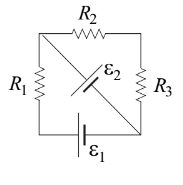# Problem: The circuit shown in the figure has resistors R1 = 10 Ω, R2 = 20 Ω, R3 = 30 Ω, ε1 = 10 V, ε2 = 20 V. What is the current through resistor R1? (1) 1 A (2) 2 A (3) 1.5 A (4) 0.5 A (5) 0.67 A

🤓 Based on our data, we think this question is relevant for Professor Muller's class at USF.

###### Problem Details

The circuit shown in the figure has resistors R1 = 10 Ω, R2 = 20 Ω, R3 = 30 Ω, ε1 = 10 V, ε2 = 20 V. What is the current through resistor R1?

(1) 1 A

(2) 2 A

(3) 1.5 A

(4) 0.5 A

(5) 0.67 A